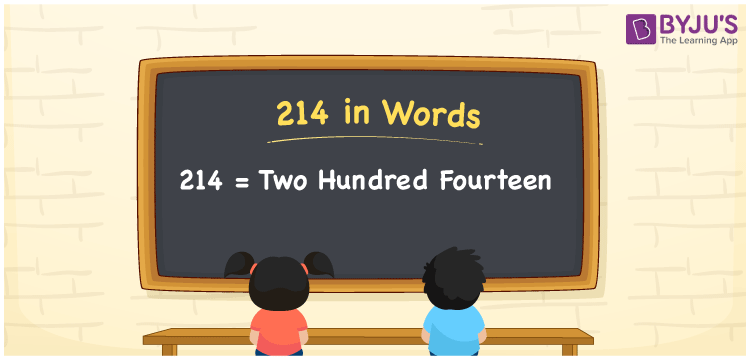# 214 in words

214 in words is written as Two Hundred and Fourteen. 214 represents the count or value. The article on Counting Numbers can give you an idea about count or counting. The number 214 is a 3 digit number that is used in expressions related to money, days, distance, length, weight and so on. Let us consider an example for 214. “I need to attend school for Two Hundred and Fourteen days in this academic year.” Another example is “This apartment complex has a parking space to park Two Hundred and Fourteen cars”

 214 in words Two Hundred and Fourteen Two Hundred and Fourteen in Numbers 214

## 214 in English Words## How to Write 214 in Words?

We can convert 214 to words using a place value chart. The number 214 has 3 digits, so let’s make a chart that shows the place value up to 3 digits.

 Hundreds Tens Ones 2 1 4

Thus, we can write the expanded form as:

2 × Hundred + 1 × Ten + 4 × One

= 2 × 100 + 1 × 10 + 4 × 1

= 214

= Two Hundred and Fourteen.

214 is the natural number that is succeeded by 213 and preceded by 215.

214 in words – Two Hundred and Fourteen.

Is 214 an odd number? – No.

Is 214 an even number? – Yes.

Is 214 a perfect square number? – No.

Is 214 a perfect cube number? – No.

Is 214 a prime number? – No.

Is 214 a composite number? – Yes.

## Solved Example

1. Write the number 214 in expanded form

Solution: 2 × 100 + 1 × 10 + 4 × 1

We can write 214 = 200 + 10 + 4

= 2 × 100 + 1 × 10 + 4 × 1.

## Frequently Asked Questions on 214 in words

Q1

### How to write the number 214 in words?

214 in words is written as Two Hundred and Fourteen.
Q2

### State True or False. 214 is divisible by 3?

False. 214 is not divisible by 3.
Q3

### Is 214 a perfect cube number?

No. 214 is not a perfect cube number.